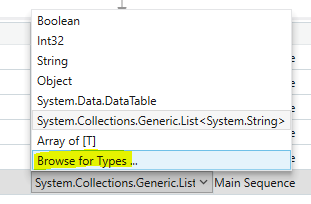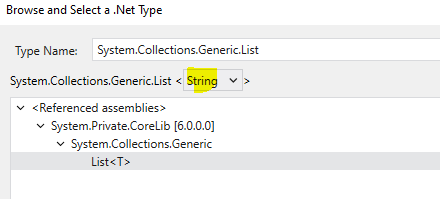# Hi i want solution

in if else condition i want to compair name in data table but i have number of name and add one by one so what i do

Can you put the below condition in the if activity and try.

**

Ename(function(x) x.ToString.Equals(CurrentRow(“Name”).ToString)).Any

**

1 Like

If you are trying to compare a value from the datatable with a list of values, then you would have to do the following corrections :

1. Declare the variable `Ename` as a List of String and assign the Default value like below :
``````new List(Of String) from {"<<me>>","Abdul Wajid",...}  //Fill the required data
``````
1. Then, In the If Condition, you would need to use the below Expression :
``````Ename.Contains(CurrentRow("Name").ToString)
``````

Let us know if you are having difficulties in performing the above or if you do not get the expected output.

1 Like

sir exp does not satisfied

sir where to create list and how to apply list

It is as mentioned above, When Browsing for Types, You would need to find the `System.Collections.Generic.List` and Select `String` like shown below :After Selection, you can follow the post from above for assigning default values.

@Shubham_Bidwai ,
It was not initialised as mentioned above . Do Check and let us know after correction.

i assign the Ename but also shows value of string can not be converted into list of string

You would need to append the below at the beginning of the array data :

``````new List(Of String) from
``````

what i assign i assign the Ename and after that change the type that is list

i did not understand plz briefly explain i am new in Ui path

Could you maybe show us Screenshots of the implementation done by you :

Check the image above if you have also performed the same.

gone error thank you so much

Could you let us know what are you trying to perform ?

You’re initial query was with comparison of datatable values. Is the Comparison happening properly ?

actuly my requrement is to ectract data from one table okay and in that table some emp are missing to fill there work that emp status is not updated is return in excel sheet
its my req
my step after table extraction
write range
read range and store in dt
read range and store in other db
clear 2nd data table
for each row
if condition
curentrow(“Name”)=list of emp
add data row in the form of arrey
i will send you screen shot also plz check and give me guidance about i